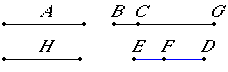# Proposition 85

To find the first apotome.

Set out a rational straight line, and let BG be commensurable in length with A. Then BG is also rational.

Set out two square numbers DE and EF, and let their difference FD not be square. Then ED does not have to DF the ratio which a square number has to a square number.

Let it be contrived that ED is to DF as the square on BG is to the square on GC. Then the square on BG is commensurable with the square on GC.

But the square on BG is rational, therefore the square on GC is also rational. Therefore GC is also rational.X.9

Since ED does not have to DF the ratio which a square number has to a square number, therefore neither has the square on BG to the square on GC the ratio which a square number has to a square number. Therefore BG is incommensurable in length with GC.

X.73

And both are rational, therefore BG and GC are rational straight lines commensurable in square only. Therefore BC is an apotome.

I say next that it is also a first apotome.

Let the square on H be that by which the square on BG is greater than the square on GC.

V.19,Cor.

Now since ED is to FD as the square on BG is to the square on GC, therefore, in conversion, as DE is to EF as the square on GB is to the square on H.

X.9

But DE has to EF the ratio which a square number has to a square number, for each is square, therefore the square on GB also has to the square on H the ratio which a square number has to a square number. Therefore BG is commensurable in length with H.

And the square on BG is greater than the square on GC by the square on H, therefore the square on BG is greater than the square on GC by the square on a straight line commensurable in length with BG.

And the whole BG is commensurable in length with the rational straight line A set out.

X.Def.III.2

Therefore BC is a first apotome. Therefore the first apotome BC has been found.

Q.E.F.

## Guide

This proposition is not used in the rest of the Elements.